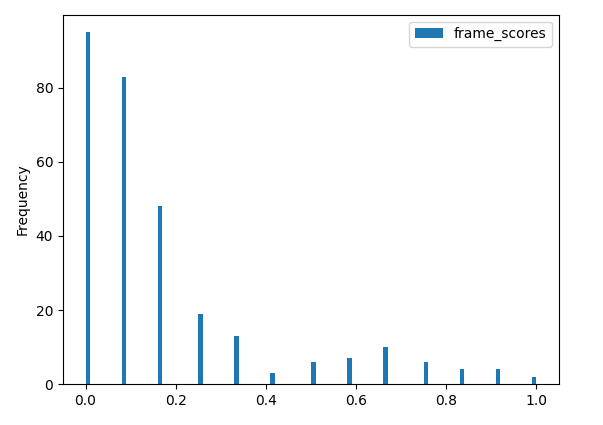# Model on regression task not converging

I have a FFN as follows a to do regression task given x

``````class MLP(nn.Module):
def __init__(self,input_dim, hidden_dim, output_dim, num_layers):
super(MLP,self,).__init__()
self.num_layers = num_layers
self.hidden = nn.Linear(input_dim, hidden_dim)
self.out = nn.Linear(hidden_dim, 1)
self.norm = nn.LayerNorm(hidden_dim)
self.dorp50 = nn.Dropout(0.5)

def forward(self,x):
x = self.norm(x)
x = self.hidden(x)
x = F.relu(x)
x = F.dropout(x)
x = self.norm(x)

x = self.out(x)
x  = F.Sigmoid(x)
return x
``````

The input the FFN is (bs,seq_len,d_model) ie. (1,1518,1024).
The output of the FNN is (bs,seq_len,d_model) ie (1,1518,1).
I trying to get a score between 0-1 for every time step.

The target is of shape (1518), a score between [0-1]. Baically a score for every time step.
This is what the distribution of the targets look likeAs you can see that the targets for most of the time stamps are mostly 0.

I am using MSELoss(). The problem is that the FFN, learns to predict all 0 as output and gets away with having a very low loss. Because it’s predicted 0 and we are using sigmoid, this leads to the gradient to be 0. Thus, the ratio of the FFN layer weights to update is 0. Hence, the model stops learning.

Is there something I am missing? I am aware that GANs generators use sigmoid to regress pixel value then use MSELoss, how come they don’t suffere from the problem I am suffering?

I am been working to debug this model for the past 2 days by now,I hope someone more knowledgeable than me can shine a light on this.

Thanks

You could try to remove the `sigmoid`, if you think it might kill the gradients.
If that doesn’t help, a good debugging step is to try to overfit a small dataset first (e.g. just take 10 samples) by playing around with some hyperparameters and make sure your model and training routine is able to do so.
Once this is done, you could scale up the use case again by using more data.
If your model isn’t able to overfit a tiny dataset, then there might be a real bug in the code (e.g. you might have forgotten to zero out the gradients) or the model is just unable to learn the distribution (which I don’t think would be the case given your explanation of the use case).